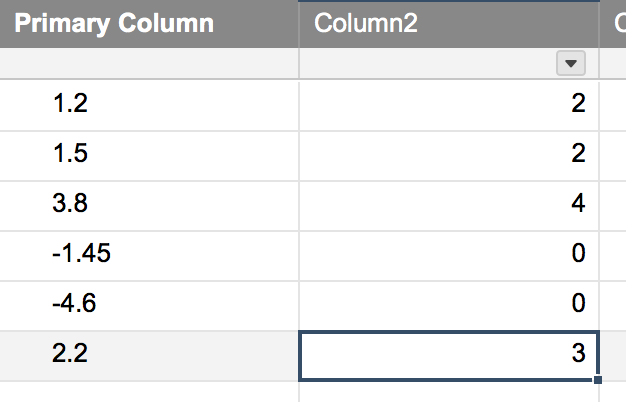# RoundUp Decimal Issues...

Trying to get a formula where any decimal number above 1 will round the next highest whole number (examples: .33 will round to 1, 1.33 will round to 2, 3.23 will round to 4, etc.).  I also need if the formula = a negative it displays a 0 as opposed to -4.

current formula using and somewhat working

=MAX(IF([Paper Towels]2 / [Paper Towels]1 - [Paper Towels]3 > 0.4, [Paper Towels]2 / [Paper Towels]1 - [Paper Towels]3 + 0.4, [Paper Towels]2 / [Paper Towels]1 - [Paper Towels]3), 0)

current Data: Paper Towels2 = 40, Paper Towels1 = 30, Paper Towels3 = 1

40(paper towel 2) / 30 (paper towel 1) = 1.33

1.33 - 1 (paper towel 3) = .33

I need .33 to round to 1 and I'm stuck.  tried the if formula with adding .04 to get it over but not working correctly.

Thanks for any help

Tags:

=IF([Primary Column]1 < 0, 0, INT([Primary Column]1) + 1)

Image is what it returns.• Well done jk. An elegant solution.

• Agreed! Worked like a charm.

• Thank you! I am glad it worked out.

## Help Article Resources

Want to practice working with formulas directly in Smartsheet?

Check out the Formula Handbook template!